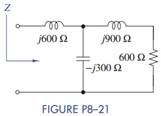### Create an Account

Home / Questions / A certain RLC series load has a ZL 100 j999 Ω when excited by a 1-krad=s source and a ...

# A certain RLC series load has a ZL 100 j999 Ω when excited by a 1-krad=s source and a ZL = 100 + j90 Ω when driven by a 100-krad=s source Find the values of R L and C 2 Find the equivalent

A certain RLC series load has a ZL = 100 – j999 Ω when excited by a 1-krad=s source and a ZL = 100 + j90 Ω when driven by a 100-krad=s source. Find the values of R, L, and C.

2. Find the equivalent impedance Z in Figure P8–21. If ω = 150 krad/s what two elements (R, L, and/or C) could be used to replace the phasor circuit?Jun 15 2020 View more View LessSubscribe To Get Solution